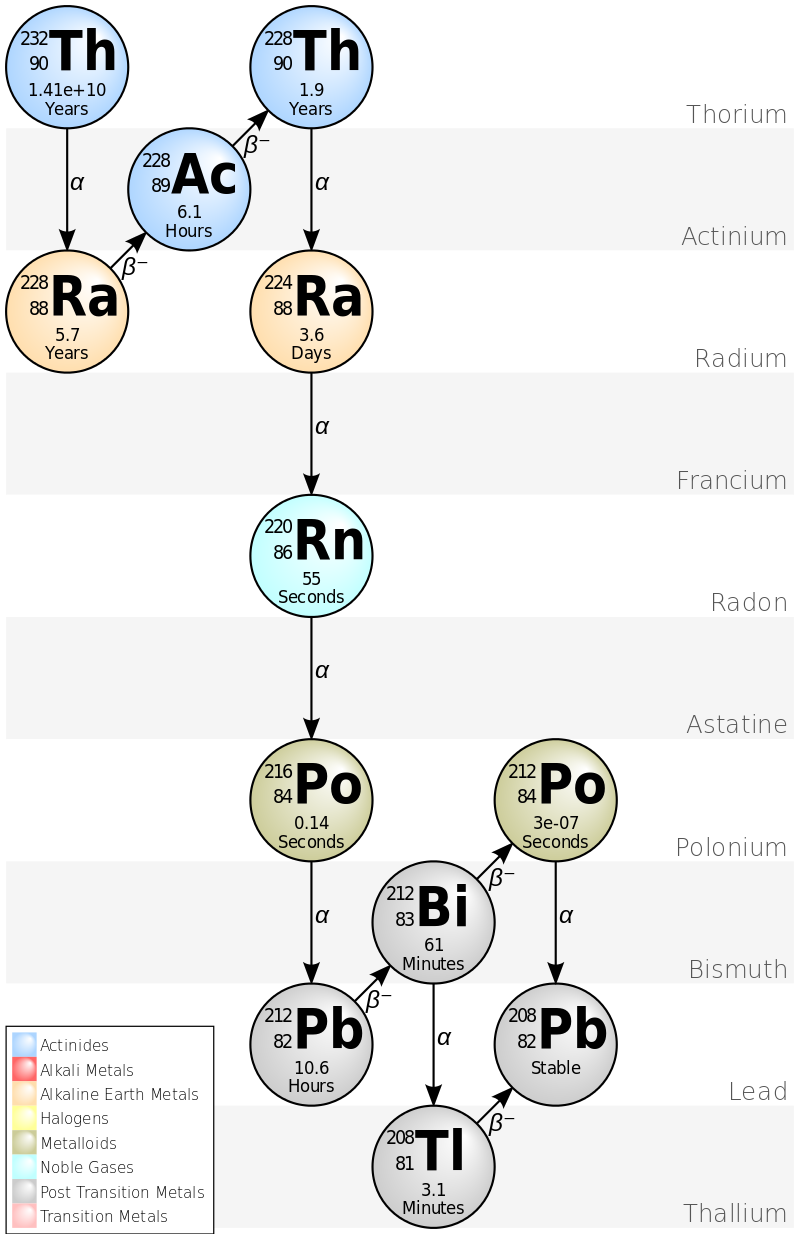• $\alpha$ 射线
高速运动的 $\alpha$ 粒子（氦核）。电离作用大，贯穿本领小。
• $\beta$ 射线
高度运动的电子，电离作用较小，贯穿本领较大。
• $\gamma$ 射线
高频光子，电离作用小，贯穿本领大。

## 放射性衰变的基本规律

### 放射性衰变的种类

• $\alpha$ 放射性
$\alpha$ 衰变
放出带正电的氦核

$\mathrm{^A_ZX}\rightarrow \mathrm{_{Z-2}^{A-4}Y} + \mathrm{^4_2He}$

• $\beta$ 放射性

• $\beta$ 衰变
放出电子

$\mathrm{^A_ZX}\rightarrow \mathrm{_{Z+1}^{A}Y} + \mathrm{e^-}$

• $\beta^+$ 衰变
放出正电子

$\mathrm{^A_ZX}\rightarrow \mathrm{_{Z-1}^{A}Y} + \mathrm{e^+}$

• 轨道电子俘获 Electron capture
内层电子被原子核俘获

$\mathrm{^A_ZX}+ \mathrm{e^-}\rightarrow \mathrm{_{Z-1}^{A}Y}$

• $\gamma$ 放射性
$\gamma$ 跃迁 （来自与核子内部能级的跃迁）

### 放射性衰变的规律

$\frac{dN}{dt} = -\lambda N$

$N(t) = N_0 e^{-\lambda t}$

• 放射性活度
当前时刻的衰变速率。

$A \equiv - \frac{dN}{dt} =\lambda N = \lambda N_0 e^{-\lambda t}$

• $\mathrm{Ci}$ 居里

$1 \mathrm{Ci} = 3.7\times 10^{10} s^{-1}$

• $\mathrm{Bq}$ 贝克勒尔

$1 \mathrm{Bq} = 1\mathrm{s}^{-1}$

• 比活度：单位质量放射源的放射性活度。
• 半衰期
一般放射性原子核衰变所需要的时间。

$T_{1/2} = \frac{\mathrm{ln}2}{\lambda}$

• 平均寿命
放射性原子核平均生存时间。

$\tau = \frac{1}{\lambda}$

### 递次衰变规律\left\{\begin{aligned} & \frac{dN_1}{dt} = -\lambda_1 N_1 \\ & \frac{dN_2}{dt} = \lambda_1 N_1-\lambda_2 N_2 \\ & \frac{dN_3}{dt} = \lambda_2 N_2\\ \end{aligned}\right.

\begin{aligned} & N_1 = N e^{-\lambda_1 t} \\ & N_2 = \frac{\lambda_1}{\lambda_2-\lambda_1}N (e^{-\lambda_1 t}-e^{-\lambda_2 t} ) \\ & N_3 = \frac{\lambda_1\lambda_2}{\lambda_2-\lambda_1}N[ \frac{1}{\lambda_1}(1-e^{-\lambda_1 t}) - \frac{1}{\lambda_2}(1-e^{-\lambda_2 t}) ] \end{aligned}

$N_3 = N (h_1e^{-\lambda_1t} + h_2e^{-\lambda_2t} + h_3e^{-\lambda_3t})$

\begin{aligned} h_1 &= \frac{\lambda_1\lambda_2}{(\lambda_2-\lambda_1)(\lambda_3-\lambda_1)} \\ h_2 &= \frac{\lambda_1\lambda_2}{(\lambda_1-\lambda_2)(\lambda_3-\lambda_2)} \\ h_3 &= \frac{\lambda_1\lambda_2}{(\lambda_1-\lambda_3)(\lambda_2-\lambda_3)} \\ \end{aligned}

$N_n(t) = N\sum_{i=1}^{n}h_ie^{-\lambda_i t},\quad h_i = \frac{\prod_{j=1}^{n-1}\lambda_j}{\prod_{j\neq i}^{n}(\lambda_j-\lambda_i)}$

## 放射性平衡

• 暂时平衡
母体的半衰期比子体的半衰期长，$\lambda_1<\lambda_2$。在观察时间内可以看出母体放射性的变化，最终母体与子体数量成固定比例，此后子体与母体的半衰期相同。

• 长期平衡
母体的半衰期远远长于子体的半衰期，$\lambda_1\ll\lambda_2$。在观察时间内母体放射性无变化，最子体的放射性也不会变化。

• 不成平衡
母体的半衰期小于子体的半衰期，$\lambda_1>\lambda_2$。母体的放射性迅速衰减，子体的放射性先增大后减少，最终按照自身的衰变规律进行。

• 钍系 $4n$
• 铀系 $4n+2$
• 锕系 $4n+3$

• 镎系 $4n+1$

## 放射性的生长

• 通过 反应堆 制备：

产量大，成本低。产物为丰中子核素，具有 $\beta^{-}$ 放射性。

• 利用强中子流来照射靶核，靶核俘获中子生成放射性核
• 利用中子引起重核裂变，从裂变碎片中提取放射性核素
• 通过 加速器 制备

产物往往为缺中子核，具有 $\beta^{+}$ 衰变或 EC，寿命较短。

• 带电粒子的核反应